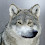## Friday, February 14, 2014

### The Ivy League Schools: 33, 55, 555 Numerology and Beyond

The Ivy League consists of eight schools in the North Eastern Region of the United States.  Each Ivy League School is located within a state that has very special numerology properties that were purposefully included in their names and important dates by the founders.  The eight schools are as follows:
1. Brown University - Providence, Rhode Island (1764)
• Brown University = 81; 17+64 = 81; Wizard = 81
2. Columbia University  - New York, New York (1754)
3. Cornell University - Ithaca, New York (1865)
4. Dartmouth College - Hanover, New Hampshire (1769)
5. Harvard University - Cambridge, Massachusetts (1636)
6. Princeton University - Princeton, New Jersey (1746)
7. University of Pennsylvania - Philadelphia, Pennsylvania (1740)
8. Yale University - New Haven, Connecticut (1701)
Seven of the eight schools were founded during the Colonial Period of the Nation, with the one exception being Cornell, which was founded on April 27, 1865.
• 4/27/1865 = 4+2+7+1+8+6+5 = 33
• 4/27/65 = 4+27+65 = 96
All seven of the original schools were established before the American Revolution.  Ivy League endowments range from \$2.2 billion annually at Brown, to \$33-billion at Yale.  The eight schools field a combined 33 sports; 17 by men and 16 by women.

The first time the group of colleges was ever referred to as the Ivy League, was by sports writer Stanley Woodward of the New York Tribute, who on October 14, 1933, wrote the following- "A portion of our eastern Ivy colleges are meeting little fellow another Saturday before plunging into the strife and the turmoil."  Thus the term Ive League, comes from the year '33.

Let us continue by examining the special numerology of the city and state each campus is located in.  As we move through this, you'll notice a lot of 33, 55 and 555; that is because those number sequences are important in numerology and to the founders of this country, as well as secret societies that keep the traditions alive even today.
• Brown University - Providence, Rhode Island (1764)
• Rhode Island is the thirteenth colony and was established 5/29/1790
• 5/29/1790 = 5+2+9+1+7+9+0 = 33
• Rhode Island = 9+8+6+4+5+9+1+3+1+5+4 = 55 = 10 = 1
• If you sum every number, one through ten, you also get 55, which is why ten is the god number in numerology, because it is a reflection of itself.
• Providence = 7+9+6+4+9+4+5+5+3+5 = 57 = 12 = 3
• Notice the coded 55 in Providence
• Brown = 2+9+6+5+5 = 27 = 9
• Notice the coded 55 in Brown
• Columbia University  - New York, New York (1754)
• Establishment date unknown...
• Columbia = 3+6+3+3+4+2+9+1 = 31 = 4
• University = 3+5+9+4+5+9+1+9+2+7 = 54/63
• Notice the coded 33 within Columbia, and the sum of 31 and 4, representing foundation
• 31 to 33 are the degrees of Freemasonry
• Cornell University - Ithaca, New York (1865)
• Ithaca = 9+2+8+1+3+1 = 24 = 6
• Cornell = 3+6+9+5+5+3+3 = 34 = 7
• Notice the coded 69, 55 and 33
• The school was established April 27, 1865
• 4/27/1865 = 4+2+7+1+8+6+5 = 33
• 4/27/1865 = 4+27+65 = 96
• Dartmouth College - Hanover, New Hampshire (1769)
• 12/13/1769
• Dartmouth = 4+1+9+2+4+6+3+2+8 = 39
• Hanover = 8+1+5+6+4+5+9 = 38 = 11 = 2
• New Hampshire = 5+5+5+8+1+4+7+1+8+9+9+5 = 67 = 13 = 4
• Notice the coded 555
• Harvard University - Cambridge, Massachusetts (1636)
• Harvard = 8+1+9+4+1+9+4 = 36 = 9
• Cambridge = 3+1+4+2+9+9+4+7+5 = 44
• Massachusetts = 4+1+1+1+1+3+8+3+1+5+2+2+1 = 33
• Notice the coded 1111, 22 and sum of 33
• The master numbers are 11, 22 and 33
• Princeton University - Princeton, New Jersey (1746)
• Princeton = 7+9+9+5+3+5+2+6+5 = 51 = 6
• New Jersey = 5+5+5+1+5+9+1+5+7 = 43 = 7
• Notice the coded 555
• University of Pennsylvania - Philadelphia, Pennsylvania (1740)
• Pennsylvania = 7+5+5+5+1+7+3+4+1+5+9+1 = 53 = 8
• Notice the coded 555
• Philadelphia = 7+8+9+3+1+4+5+3+7+8+9+1 = 65 = 11 = 2
• Philadelphia is the original capital of the Nation, and is named after a town in the Bible
• Yale University - New Haven, Connecticut (1701)
• Yale = 7+1+3+5 = 16 = 7
• New Haven = 5+5+5+8+1+4+5+5 = 38 = 11
• Notice the coded 555 and 55
• Connecticut = 3+6+5+5+5+3+2+9+3+3+2 = 46 = 10 = 1
• Notice the coded 555 and 33
To close, we'll examine some interesting facts pertaining to each school, starting with Brown, which began as the first Colonial Baptist College.  Columbia University is known for awarding the Pulitzer Prize and was the first college to award an M.D. Degree in the United States.  Cornell University came to be when Senator Ezra Cornell donated 300 acres of land to start the school.  Dartmouth College was founded by Eleazer Wheelock, with the hope of educating Natives.  Harvard University is the oldest institution of higher learning in the United States and is the alma matter of more than sixty billionaires and 335 Rhodes Scholars (The numerology of Rhodes is 33).  Princeton is home of the Woodrow Wilson School of Public and International Affairs.  Yale is the home of Skull and Bones, and is located in the same town that the Busy Dynasty is from.
• Rhodes = 9+8+6+4+5+1 = 33
• As in Rhodes Scholarship, created by Oxford University
• Oxford = 666694
• Woodrow Wilson's Birthday = 12/28/1856 = 33
• Woodrow Wilson was the first President to have a birthday/life number of 33.

1.2.